Refer to our Texas Go Math Grade 8 Answer Key Pdf to score good marks in the exams. Test yourself by practicing the problems from Texas Go Math Grade 8 Unit 4 Study Guide Review Answer Key.

Module 11 Equations and Inequalities with the Variable on Both Sides

Solve. (Lessons 11.1. 11.2, 11.3, 11.4)

Question 1.
13 – 6y = 8y
13 = 8y + 6y
14y = 13
y = 13/14
y = 0.9

$$\frac{1}{5}$$x + 5 = 19 – $$\frac{1}{2}$$x
Determine the least common multiple of the denominators: LCM(5, 2) = 10.
Then, multiply both sides of the equation by the LCM to eliminate the fraction.
$$\frac{1}{5}$$x + 5 = 19 – $$\frac{1}{2}$$x
10($$\frac{1}{5}$$x + 5) = 10(19 – $$\frac{1}{2}$$x)
2x + 50 = 190 – 5x
(Subtract 50 from both sides.) 2x = 140 – 5x
(Add 5x to both sides.) 7x = 140
(Divide both sides by 7) x = 20
x = 20

Question 3.
7.3t + 22 ≤ 2.1t – 22.2
Multiply both sides of the equation by 10. Multiplying by 10 clears the equation of decimals
7.3t + 22 = 2.1t – 22.2
73t + 220 = 21t – 222
(Subtract 220 from each side) 73t = 21t – 442
(Subtract 21t from each side.) 52t = -442
(Divide by 52) y = -8.5
y = -8.5

Question 4.
7 – 45z < 5z + 13
Given,
7 – 45z < 5z + 13
7 – 13 < 5z + 45z
-6 < 50z
50z > -6
z > -3/25

Question 5.
1.4 + $$\frac{2}{5}$$e ≥ $$\frac{3}{15}$$e – 0.8
Determine the least common multiple of the denominators: LCM(5, 15) = 15.
Then, multiply both sides of the equation by the LCM to eliminate the fraction.
1.4 + $$\frac{2}{5}$$e ≥ $$\frac{3}{15}$$e – 0.8
15(1.4 + $$\frac{2}{5}$$e) = 15($$\frac{3}{15}$$e – 0.8)
21 + 6e = 3e – 12
(Subtract 3e from both sides.) 21 + 3e = – 12
(Subtract 21 from both sides.) 3e = -33
(Divide both sides by 3) e = -11
e = 11

Question 6.
0.75x – 6.5 = -0.5 – 0.25x
Given,
0.75x – 6.5 = -0.5 – 0.25x
0.75x + 0.25x = -0.5 + 6.5
x = 6
So, the value of x is 6.

Write a real-world situation that could be modeled by the equation 650 + 10m = 60m + 400. (Lesson 11.1)
A handyman charges 650 dollars plus 10 dollars per hour for house painting. A painter charges 400 dollars plus 60 dollars per hour. How many hours would a job have to take for the handyman’s fee and the painter’s fee to be the same?

Question 8.
John is trying to decide which carpeting company to use to put carpet in his living room. Carla’s Carpeting charges $45 plus$5.50 per square foot. Fred’s Flooring charges $195 plus$4.25 per square foot. For what size room is Carla’s Carpeting cheaper than Fred’s Flooring? (Lesson 11.3)
45 + 5.50f = 195 + 4.25f

Question 1.
CARRERS IN MATH Hydraulic Engineer A hydraulic engineer studies the pressure in a particular fluid. The pressure is equal to the atmospheric pressure 101 kN/m plus 8 kN/m for every meter below the surface, where kN/m is kilonewtons per meter, a unit of pressure.
a. Write an expression for the pressure at a depth of d, meters below the liquid surface.
where P is the pressure at d1 below the surface
P = 8d1 + 101

b. Write and solve an equation to find the depth at which the pressure is 200 kN/m.
When P = 200 kN/m
200 = 8d1 + 101
Subtract 101 from both sides
200 – 101 = 8d1 + 101 – 101
8d1 = 99
Divide both sides by 8
d1 = 12.375

c. The hydraulic engineer alters the density of the fluid so that the pressure at depth d2 below the surface is atmospheric pressure 101 kN/m plus 9 kN/m for every meter below the surface. Write an expression for the pressure at depth d2.
where P is the pressure at d2 below the surface
P = 9d2 + 101

d. If the pressure at depth d1 in the first fluid is equal to the pressure at depth d2 in the second fluid, what is the relationship between d1 and d2? Explain how you found your answer.
When pressure is equal the equations from a and c should be set equal
8d1 + 101 = 9d2 + 101
Subtract 101 from both sides
8d1 + 101 – 101 = 9d2 + 101 – 101
8d1 = 9d2
Divide both sides by 8
d1 = $$\frac{9}{8}$$ d2

Texas Go Math Grade 8 Unit 4 Mixed Review Texas Test Prep Answer Key

Selected Response

Question 1.
Ricardo and John start swimming from the same location. Ricardo starts 15 seconds before John and swims at a rate of 3 feet per second. John swims at a rate of 4 feet per second in the same direction as Ricardo. Which equation could you solve to find how long it will take John to catch up with Ricardo?
(A) 4t + 3 = 3t
(B) 4t + 60 = 3t
(C) 3t + 3 = 4t
(D) 3t + 45 = 4t
(D) 3t + 45 = 4t

Explanation:
If v shows at which rate Ricardo and John swim, d is the distance between two locations and t is their time, then we use the formula for speed:
v = $$\frac{d}{t}$$
t ∙ (v) = t ∙ ($$\frac{d}{t}$$) (Multiply both sides by t)
t ∙ (v) = d
John swims at a rate of 4 feet per second
d1 = 4t (for v = 4 ft/s)
Ricardo swims 15 seconds more than John and swims at a rate of 3 feet per second.
d2 = 3(15 + t) = 45 + 3t
d1 = d2 (for v = 3 ft/s and his time is t + 15)
4t = 45 + 3t

Question 2.
Gina and Rhonda work for different real estate agencies. Gina earns a monthly salary of $5,000 plus a 6% commission on her sales. Rhonda earns a monthly salary of$6,500 plus a 4% commission on her sales. How much must each sell to earn the same amount in a month?
(A) $1,500 (B)$15,000
(C) $75,000 (D)$750,000
(C) $75,000 Explanation: We write an expression representing the monthly earnings of Gina. Let’s denote the number of sales by s. 5000 + 0.06s We write an expression representing the monthly earnings of Rhonda. Let’s denote the number of sales by s. 6500 + 0.04s We write an equation that can be solved to find how much must each sell to earn the same amount in a month. 5000 + 0.06s = 6500 + 0.04s 100 ∙ (5000 + 0.06s) = (6500 + 0.04s) ∙ 100 500000 + 6s = 650000 + 4s 500000 + 6s – 4s = 650000 + 4s – 4s 500000 + 2s = 650000 500000 + 2s – 500000 = 650000 – 500000 2s = 150000 s = $$\frac{150000}{2}$$ s = 75000 Each of them should sell$75000 to earn the same amount in a month.

Which is the measure of ∠BAC in the triangle below?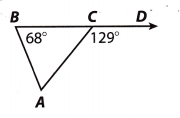(A) 51°
(B) 61°
(C) 68°
(D) 71°
∠ACD = 129°
Sum of angles = 180°
∠BAC = 180° – 129°
∠BAC = 51°
Thus the correct answer is option A.

Question 4.
A movie theater has two membership plans. Under Plan A you pay $6 a month plus$4 for each ticket you buy. Under Plan B you pay $18 a month plus$2 for each ticket you buy. Which inequality represents the situation when Plan B becomes less expensive than Plan A?
(A) x > 2
(B) x< 2
(C) x > 6
(D) x < 6
Plan A you pay per month = $6 +$4 for each ticket.
Plan B you pay per month = $18 +$2 for each ticket.
$18 +$2x < $6 +$4x
$18 -$6 < $4x –$2x
$12 <$2x
x < $12/$2
x < 6
Option B is the correct answer.

Question 5.
Which inequality represents the solution to 1.75x + 3.5 > 3.25x – 8.5?
(A) x > 8
(B) x < 8
(C) x > 3.3
(D) x < 3.3
1.75x + 3.5 > 3.25x – 8.5
3.5 + 8.5 > 3.25x – 1.75x
12 > 1.5x
x < 12/1.5
x < 8
Thus the correct answer is option B.

Question 6.
The triangle and the rectangle have the same perimeter.Find the value of x.
(A) 2
(B) 10
(C) 18
(D) 24
(A) 2

Explanation:
The perimeter of the rectangle is
Pr = 2l + 2w
Pr = 2(x + 7) + 2(2x – 1)
Pr = 2r + 14 + 4x – 2
Pr = 6x+ 12
The perimeter of the triangle is
Pt = a + b + c
Pt = (x + 5) + (x + 6) + (x + 7)
Pt = x + 5 + x + 6 + x + 7
Pt = 3x + 18
The perimeter of the rectangle is equal to the perimeter of the triangle, therefore we solve for x
Pr = Pt
6x + 12 = 3x + 18
6x + 12 – 3x = 3x + 18 – 3x
3x + 12 = 18
3x = 6
x = $$\frac{6}{3}$$
x = 2

Unit 4 Test Study Guide Congruent Triangles Answer Key Question 7.
What is the slope of the line?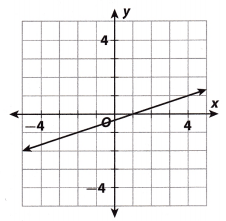(A) -3
(B) –$$\frac{1}{3}$$
(C) $$\frac{1}{3}$$
(D) 3
(C) $$\frac{1}{3}$$

Explanation:
In general, the slope of a Line is the ratio of the change in y-vaLues for a segment of the graph to the
corresponding change in x-values.
slope = $$\frac{y_{2}-y_{1}}{x_{2}-x_{1}}$$
Choosing the points (1, 0) and (4, 1) on the given Line, we substitute the x- and y- values in equation (1).
slope = $$\frac{1-0}{4-1}=\frac{1}{3}$$
slope = $$\frac{1}{3}$$

Question 8.
A square wall tile has an area of 58,800 square millimeters. Between which two measurements is the length of one side?
(A) between 24 and 25 millimeters
(B) between 76 and 77 millimeters
(C) between 242 and 243 millimeters
(D) between 766 and 767 millimeters
Answer: (C) between 242 and 243 millimeters
Explanation:
A square wall tile has an area of 58,800 square millimeters.
Area of a square = s . s
A = 58,800
s = √58800
Perfect square close to 58800 is 58564 < 58800 < 59049
= 242 <√58800 < 243
The correct answer is option C.

Gridded Response

8th Grade Unit 4 Study Guide Congruent Triangles Question 9.
For the inequality 3x – 5 ≥ 5x – 25, what is the greatest value of x that makes the statement true?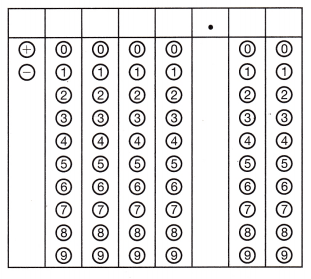3x – 5 = 5x – 25
3x – 5x = -25 + 5
-2x = -20
x = 20/2
x = 10

Hot Tips! Correct answers in gridded problems can be positive or negative. Enter the negative sign in the first column when it is appropriate. Check your work!

Question 10.
Two cars are traveling in the same direction. The first car is going 45 mi/h and the second car is going 60 mi/h. The first car left 2 hours before the second car. How many hours will it take for the second car to travel the same distance as the first car?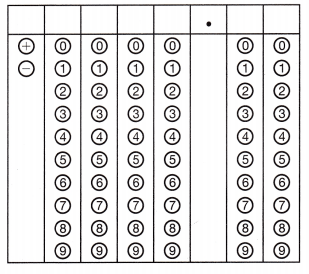Given that,
The first car is going per mi/h = 45.
The second car is going per mi/h = 60.
The first car left 2 hours before the second car.
The equation is
45(t + 2) = 60 × t
45t + 90 = 60 × t
45t + 90/t = 60
45 + 90/t = 60
90/t = 15
90 = 15t
t = 90/15
t = 6.
The second car takes 6 hours to travel the same distance as the first car.

Study Guide 8th Grade Math Unit 4 Question 11.
Mickey is ordering some clothes online. One website charges $1.25 per pound for shipping. The other website charges$0.75 per pound for shipping plus a $2 handling fee. How many pounds of clothing would Mickey need to order for the shipping costs to be the same?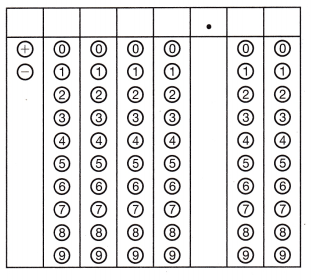Answer: One website charges for shipping =$1.25 per pound.
Other websites charge for shipping = $0.75 per pound +$2 handling fee.
$1.25x =$0.75x + $2.$1.25x – $0.75x =$2
$0.5x =$2
x = 2/0.5
x = 4 pounds.
Mickey needs 4 pounds to order for the shipping costs to be the same.

Use the puzzle to preview key vocabulary from this unit. Unscramble the circled letters to answer the riddle at the bottom of the page.1. A number that is multiplied by the variable in an algebraic expression, where the number is a fraction. (Lesson 11.2)
2. A number that is multiplied by the variable in an algebraic expression, where the number is a decimal. (Lesson 11.2)
3. A mathematical statement that two expressions are equal. (Lesson 11.1)
4. A number that is formed by repeated multiplication of the same factor, Multiply this to remove decimals from an unsolved equation. (Lesson 11.2)
5. A statement that two expressions are not equal. (Lesson 11.3)

Question.
What is the best time to divide a half-dollar between two people?